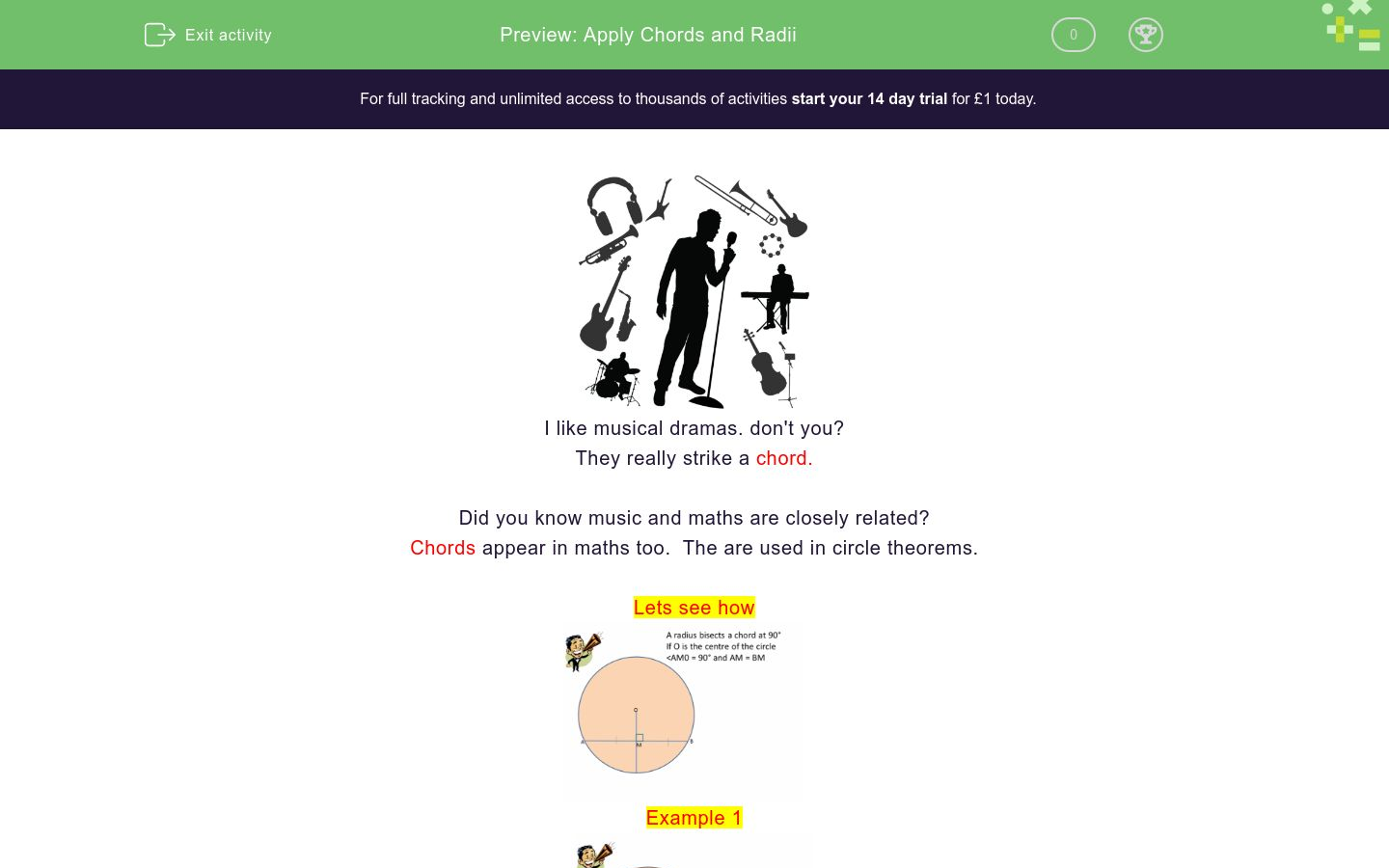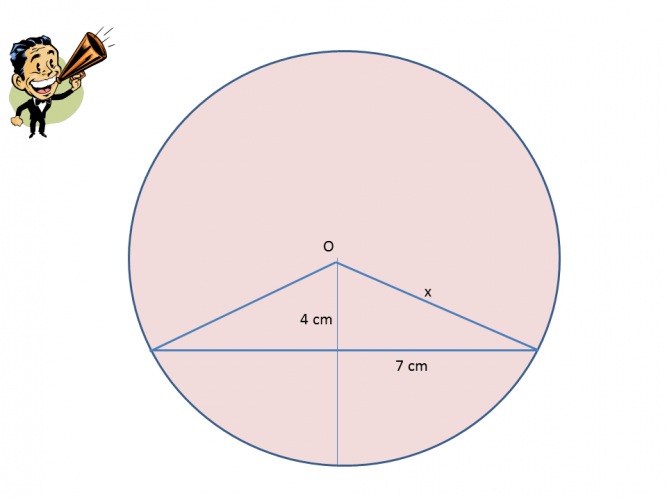### Affordable pricing from £10/month

In this worksheet, students will learn the circle theorem about chords and radii and be able to apply them.Key stage:  KS 4

GCSE Subjects:   Maths

GCSE Boards:   OCR, AQA, Eduqas, Pearson Edexcel

Curriculum topic:   Basic Geometry, Geometry and Measures

Curriculum subtopic:   Circles, Properties and Constructions

Difficulty level:### QUESTION 1 of 10I like musical dramas. don't you?

They really strike a chord.

Did you know music and maths are closely related?

Chords appear in maths too.  The are used in circle theorems.

Lets see howExample 1The rule is where the radius bisects the chord it is at right angles 90°

To find angle x we can see now that  this is a straight forward angles in a triangle add up to 180°

180 - 90 - 48 = 42°

Example 2As the chord cuts the radius at 90° we can see that a right angled triangle is formed.

We can apply Pythagoras' Theorem to find side lengths.

8 cm² - 6 cm² = 28 cm²

√ 28 = 5.29 cm

A shorter side of the triangle is being found hence the subtraction in Pythagoras' Theorem.

Over to you..Find the value of x.90°

63°

153°

27°38° 52° 88° 90° Value of x Value of yWhat is the value of x?

15°

30°

75°

90°15°

30°

75°

90°Find the value of x.

15°

30°

75°

90°Find the value of x.What is the value of x

8.06 cm

5.74 cm

4.68 cm

6.72 cmFind the value of x.

• Question 1Find the value of x.

38
EDDIE SAYS
The thing here is that the right angle sign is not shown in circle theorems. This doesn't matter to you as you know the chord cuts the radius at 90°. Now you just find the missing angle in a triangle. 180 - 90 - 52 = 38°
• Question 227°
EDDIE SAYS
Why do they have to make a simple angles in a triangle look so complicated? All we need to do is 180 - 90 - 63 = 27°
• Question 338° 52° 88° 90° Value of x Value of y
EDDIE SAYS
Once you find the right angle the rest is pretty straightforward. 180 - 90 - 38 = 52°
• Question 4What is the value of x?

15°
EDDIE SAYS
This is a case of applying prior knowledge. In this case solving equations. We can see that 6x + 90 = 180° Lets solve this 6x = 90 x = 15. Substitute into the triangle to check they add up to 180° 90 + 75 + 15 = 180°
• Question 5EDDIE SAYS
How did you find this one. We can see that 5x + 90 = 180° Lets solve this 5x = 90 x = 18 2x = 36° and 3x = 54° Final check 90 + 36 + 54 = 180°
• Question 6Find the value of x.

EDDIE SAYS
Because the chord cuts the radius at right angles we can apply Pythagoras' Theorem to find the missing side. He gets everywhere doesn't he. 6 cm² + 3 cm² = 45 cm² √ 45 = 6.71 cm
• Question 7Find the value of x.

12.08
EDDIE SAYS
Are you spotting these easier now? 11 cm² + 5 cm² = 146 cm² √ 146 = 12.08 cm to 2 decimal places. It is great that what you learn in maths never stands alone. Who would have thought Pythagoras, the triangle man would pop up in circles.
• Question 8EDDIE SAYS
Pythagoras is a top bloke isn't he. He can help us solve all sorts of problems. Did you spot you were finding a shorter side so therefore a subtraction. 12 cm² = 6 cm² = 108 cm² √ 108 = 10.39 cm. Job done.
• Question 9What is the value of x

8.06 cm
EDDIE SAYS
Once you can spot these this it is pretty straightforward isn't it. A triangle in a circle, no problems. 7 cm² + 4 cm² = 65 cm² √ 65 = 8.06 cm to 2 decimal places.
• Question 10Find the value of x.

9.19
EDDIE SAYS
Why did the acute angle dislike 90°? Because they are always right. Who would have thought that when you were younger, the first angle you were probably taught could help so much in your higher level work. 6.5 cm² + 6.5 cm² = 84.5 cm² √ 84.5 = 9.19 cm to 2 decimal places.
---- OR ----

Sign up for a £1 trial so you can track and measure your child's progress on this activity.

### What is EdPlace?

We're your National Curriculum aligned online education content provider helping each child succeed in English, maths and science from year 1 to GCSE. With an EdPlace account you’ll be able to track and measure progress, helping each child achieve their best. We build confidence and attainment by personalising each child’s learning at a level that suits them.

Get started